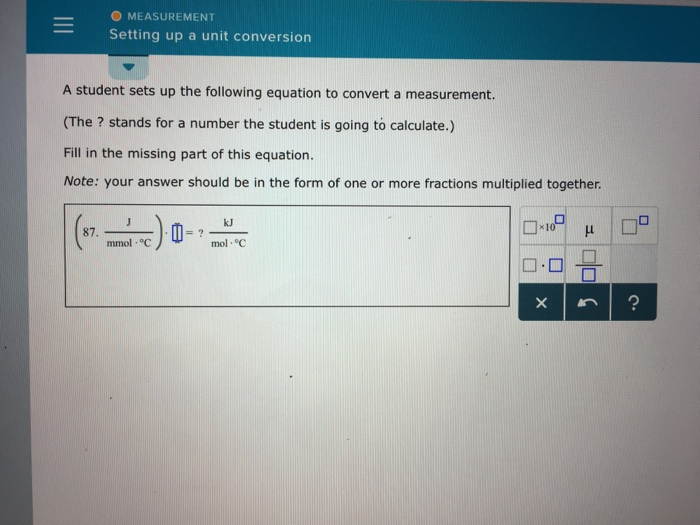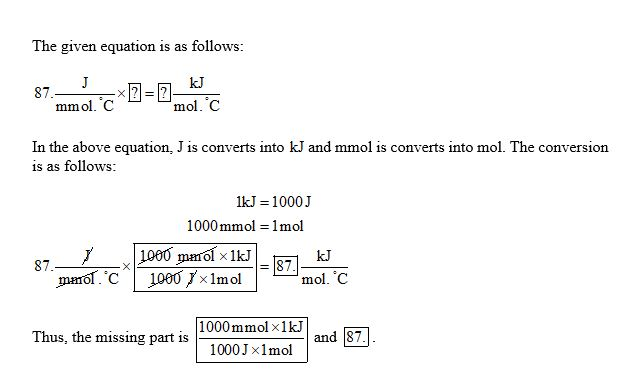# Question & Answer: A student sets up the following equation to convert a measurement. (The ? stands for a number…..A student sets up the following equation to convert a measurement. (The ? stands for a number the student is going to calculate.) Fill in the missing part of this equation. Note: your answer should be in the form of one or more fractions multiplied together.